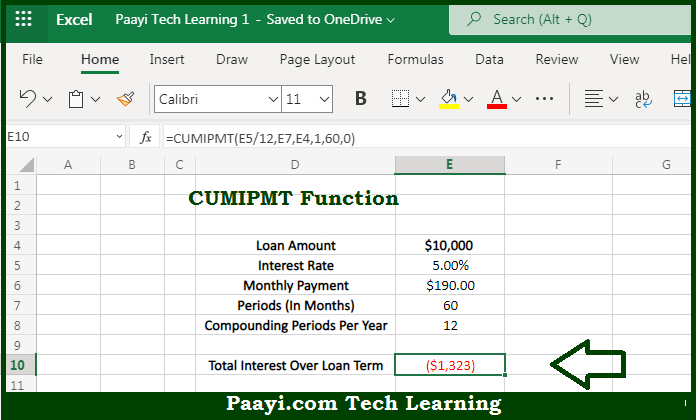# Learn How to Use Microsoft Excel CUMIPMT Function

Written by | 0 Comments | 586 Views

In this article, you will learn how to use the Microsoft Excel CUMIPMT function and its prime function in Microsoft Excel. You will also get to know the Microsoft Excel CUMIPMT function return value and syntax with the help of some examples.

Microsoft Excel CUMIPMT Function

The main purpose of the Microsoft Excel CUMIPMT function is to get the cumulative interest paid on the loan. That implies, with the help of the CUMIPMT function you can able to return the cumulative interest paid on a loan between a start period and an end period. It should be noted that you can use CUMIPMT to calculate and verify the total interest paid on a loan, or the interest paid between any two payment periods. So, with the help of the CUMIPMT function, you can able to get the cumulative interest paid on the loan.

Return Value of CUMIPMT Function

The return value will be the interest amount.

Syntax of CUMIPMT Function

=CUMIPMT(rate, nper, pv, start_period, end_period, type)

Where the arguments:

• rate: This is the interest rate of the period.
• nper: This is the total number of payments for the loan.
• pv: This is the present value or total values of all payments now.
• start_period: This is the first payment in the calculation.
• end_period: This is the last payment in the calculation.
• type: This can be understood as when payments are due. 0 = end of the period. 1 = beginning of the period.

How to Use Microsoft Excel CUMIPMT Function?So we know that Microsoft Excel CUMIPMT function you can able to get the cumulative interest paid on the loan. That implies, with the help of the CUMIPMT function you can able to return the cumulative interest paid on a loan between a start period and an end period. It should be noted that you can use CUMIPMT to calculate and verify the total interest paid on a loan, or the interest paid between any two payment periods. So, with the help of the CUMIPMT function, you can able to get the cumulative interest paid on the loan.